# Frobenius automorphism

(diff) ← Older revision | Latest revision (diff) | Newer revision → (diff)
An element of a Galois group of a special type. It plays a fundamental role in class field theory. Suppose thatis an algebraic extension of a finite field. Then the Frobenius automorphism is the automorphism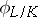defined by the formula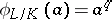for all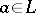, where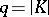(the cardinality of). If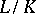is a finite extension, thengenerates the Galois group. For an infinite extension,is a topological generator of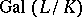. Ifand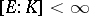, then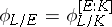.
Suppose thatis a local field with a finite residue field, and thatis an unramified extension of. Then the Frobenius automorphism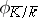of the extension of residue fields can be uniquely lifted to an automorphism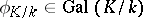, called the Frobenius automorphism of the unramified extension. Let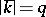, let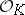be the ring of integers of, and letbe a maximal ideal in. Then the Frobenius automorphismis uniquely determined by the condition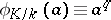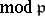for every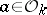. If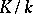is an arbitrary Galois extension of local fields, then sometimes any automorphismthat induces a Frobenius automorphism in the sense indicated above on the maximal unramified subextension ofis called a Frobenius automorphism of.
Letbe a Galois extension of global fields, let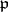be a prime ideal of, and letbe some prime ideal ofover. Suppose also that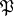is unramified inand that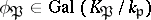is the Frobenius automorphism of the unramified extension of local fields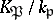. If one identifies the Galois groupwith the decomposition subgroup ofin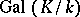, one can regardas an element of. This element is called the Frobenius automorphism corresponding to the prime ideal. Ifis a finite extension, then, according to the Chebotarev density theorem, for any automorphismthere is an infinite number of prime ideals, unramified in, such that. For an Abelian extension, the element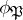depends only on. In this caseis denoted by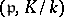and is called the Artin symbol of the prime ideal.